Chapter 1.4, Problem 1E

Chapter
Section
Textbook Problem

In Exercises 1-6, match the statement with one of the graphs (a)—(f).1. The slope of the line is zero. (a)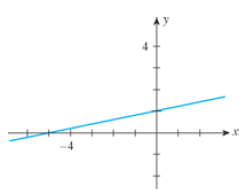(b)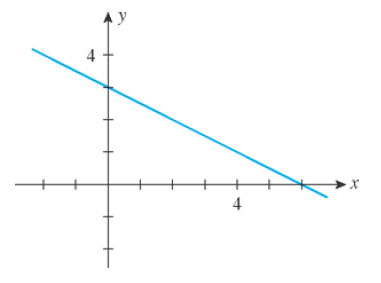(c)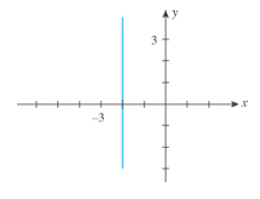(d)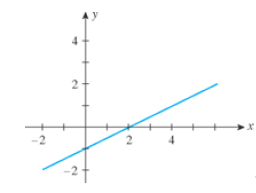(e)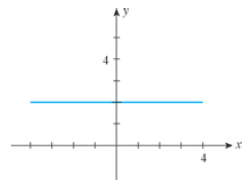(f)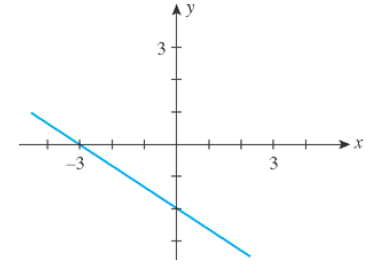To determine

To match: The graph of the line with slope as zero.

Explanation

The slope of a line is zero means the slope of a horizontal line is zero.

The equation of the line has the slope,

y=mx+b

For zero slope, the equation becomes,

y=(0)x+b

Still sussing out bartleby?

Check out a sample textbook solution.

See a sample solution

The Solution to Your Study Problems

Bartleby provides explanations to thousands of textbook problems written by our experts, many with advanced degrees!

Get Started

Use a graphing utility to graph the cost function in Example 5.

Calculus: An Applied Approach (MindTap Course List)

Convert the expressions in Exercises 6584 to power form. 23(x2+1)33(x2+1)734

Finite Mathematics and Applied Calculus (MindTap Course List)

Absolute Value Evaluate each expression. 71. (a) | ( 2) 6| (b) (13)(15)

Precalculus: Mathematics for Calculus (Standalone Book)

For the following set of scores, find the value of each expression: a. X b. (X)2 c. X2 d. (X +3)

Essentials of Statistics for The Behavioral Sciences (MindTap Course List)